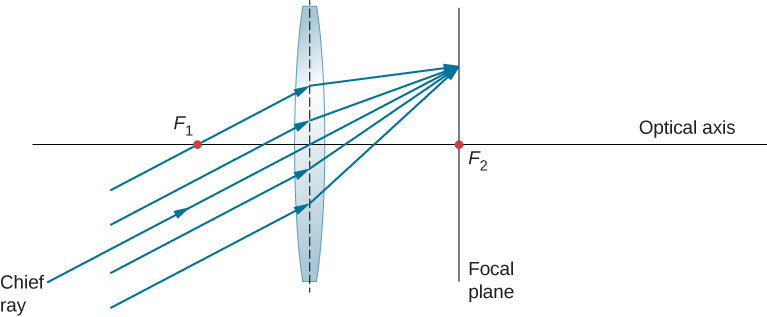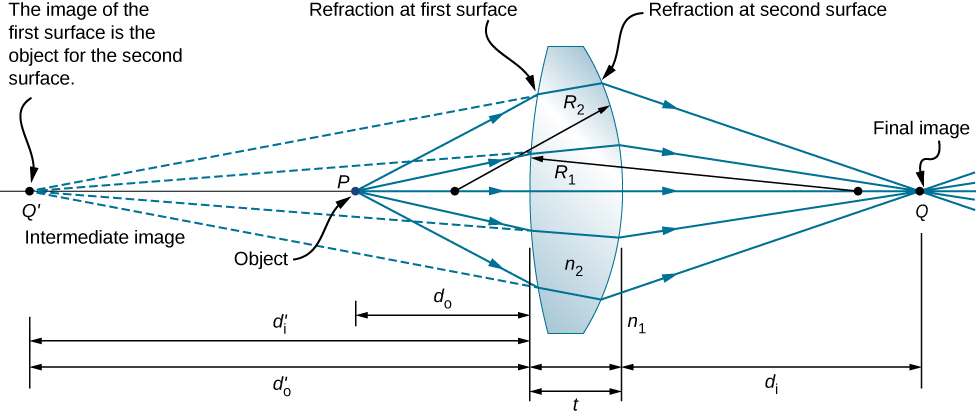# 2.4 Thin lenses  (Page 4/13)

 Page 4 / 13

## Oblique parallel rays and focal plane

We have seen that rays parallel to the optical axis are directed to the focal point of a converging lens. In the case of a diverging lens, they come out in a direction such that they appear to be coming from the focal point on the opposite side of the lens (i.e., the side from which parallel rays enter the lens). What happens to parallel rays that are not parallel to the optical axis ( [link] )? In the case of a converging lens, these rays do not converge at the focal point. Instead, they come together on another point in the plane called the focal plane    . The focal plane contains the focal point and is perpendicular to the optical axis. As shown in the figure, parallel rays focus where the ray through the center of the lens crosses the focal plane.Parallel oblique rays focus on a point in a focal plane.

## Thin-lens equation

Ray tracing allows us to get a qualitative picture of image formation. To obtain numeric information, we derive a pair of equations from a geometric analysis of ray tracing for thin lenses. These equations, called the thin-lens equation and the lens maker’s equation, allow us to quantitatively analyze thin lenses.

Consider the thick bi-convex lens shown in [link] . The index of refraction of the surrounding medium is ${n}_{1}$ (if the lens is in air, then ${n}_{1}=1.00$ ) and that of the lens is ${n}_{2}$ . The radii of curvatures of the two sides are ${R}_{1}\phantom{\rule{0.2em}{0ex}}\text{and}\phantom{\rule{0.2em}{0ex}}{R}_{2}$ . We wish to find a relation between the object distance ${d}_{\text{o}}$ , the image distance ${d}_{\text{i}}$ , and the parameters of the lens.Figure for deriving the lens maker’s equation. Here, t is the thickness of lens, n 1 is the index of refraction of the exterior medium, and n 2 is the index of refraction of the lens. We take the limit of t → 0 to obtain the formula for a thin lens.

To derive the thin-lens equation , we consider the image formed by the first refracting surface (i.e., left surface) and then use this image as the object for the second refracting surface. In the figure, the image from the first refracting surface is ${Q}^{\prime }$ , which is formed by extending backwards the rays from inside the lens (these rays result from refraction at the first surface). This is shown by the dashed lines in the figure. Notice that this image is virtual because no rays actually pass through the point ${Q}^{\prime }$ . To find the image distance ${d}_{\text{i}}^{\prime }$ corresponding to the image ${Q}^{\prime }$ , we use [link] . In this case, the object distance is ${d}_{\text{o}}$ , the image distance is ${d}_{\text{i}}^{\prime }$ , and the radius of curvature is ${R}_{1}$ . Inserting these into [link] gives

$\frac{{n}_{1}}{{d}_{\text{o}}}+\frac{{n}_{2}}{{d}_{\text{i}}^{\prime }}=\frac{{n}_{2}-{n}_{1}}{{R}_{1}}.$

The image is virtual and on the same side as the object, so ${d}_{\text{i}}^{\prime }<0$ and ${d}_{\text{o}}>0$ . The first surface is convex toward the object, so ${R}_{1}>0$ .

To find the object distance for the object Q formed by refraction from the second interface, note that the role of the indices of refraction ${n}_{1}$ and ${n}_{2}$ are interchanged in [link] . In [link] , the rays originate in the medium with index ${n}_{2}$ , whereas in [link] , the rays originate in the medium with index ${n}_{1}$ . Thus, we must interchange ${n}_{1}$ and ${n}_{2}$ in [link] . In addition, by consulting again [link] , we see that the object distance is ${d}_{\text{o}}^{\prime }$ and the image distance is ${d}_{\text{i}}$ . The radius of curvature is ${R}_{2}$ Inserting these quantities into [link] gives

what is bohrs model for hydrogen atom
hi
Tr
Hello
Youte
Hi
Nwangwu-ike
hi
Siddiquee
what is the value of speed of light
1.79×10_¹⁹ km per hour
Swagatika
what r dwarf planet
what is energy
কাজের একক কী
Jasim
কাজের একক কী
Jasim
friction ka direction Kaise pata karte hai
friction is always in the opposite of the direction of moving object
Punia
A twin paradox in the special theory of relativity arises due to.....? a) asymmetric of time only b) symmetric of time only c) only time
b) symmetric of time only
Swagatika
fundamental note of a vibrating string
every matter made up of particles and particles are also subdivided which are themselves subdivided and so on ,and the basic and smallest smallest smallest division is energy which vibrates to become particles and thats why particles have wave nature
Alvin
what are matter waves? Give some examples
according to de Broglie any matter particles by attaining the higher velocity as compared to light'ill show the wave nature and equation of wave will applicable on it but in practical life people see it is impossible however it is practicaly true and possible while looking at the earth matter at far
Manikant
a centeral part of theory of quantum mechanics example:just like a beam of light or a water wave
Swagatika
Mathematical expression of principle of relativity
given that the velocity v of wave depends on the tension f in the spring, it's length 'I' and it's mass 'm'. derive using dimension the equation of the wave
What is the importance of de-broglie's wavelength?
he related wave to matter
Zahid
at subatomic level wave and matter are associated. this refering to mass energy equivalence
Zahid
it is key of quantum
Manikant
how those weight effect a stable motion at equilibrium
how do I differentiate this equation- A sinwt with respect to t
just use the chain rule : let u =wt , the dy/dt = dy/du × du/dt : wA × cos(wt)
Jerry
I see my message got garbled , anyway use the chain rule with u= wt , etc...
Jerry
de broglie wave equation
vy beautiful equation
chandrasekhar
what is electro statics
when you consider systems consisting of fixed charges
SherlyBy OpenStaxBy OpenStaxBy David CoreyBy OpenStaxBy OpenStaxBy Laurence BailenBy OpenStaxBy OpenStaxBy OpenStaxBy OpenStax